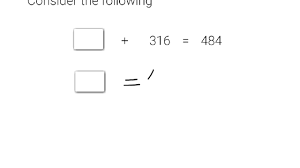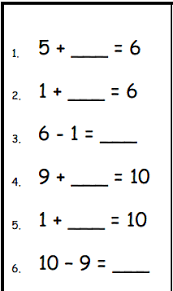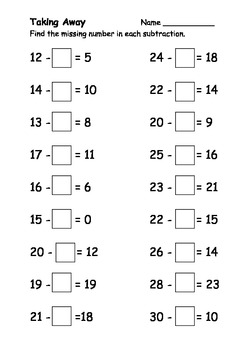# OPEN SENTENCES INVOLVING ADDITION AND SUBTRACTION

HELLO CHILDREN, WELCOME BACK TO CLASS

An open sentence can be either true or false depending on what values are used. The value we don’t know is called a variable (also called an unknown). Solving means finding a value for the variable that makes the sentence true.

Example:

A sentence that has a variable in it, such as 10 + x = 15, is called an open sentence. To solve an open sentence, replace the variable with a number that makes the sentence true. The number that makes the number sentence true is called the solution. The solution of 10 + x = 15 is the number 5.

Example 2:                                        Example 3                             Example 3::

12 + = 55                               17 = – 8                      14 =  – 9

Solution =  55 – 12 = 43                 17 + 8 = 25                           14 + 9 =

= 43                                                  so = 25                                  . So = 23ACTIVITY: Fill the blank spacesYour Opinion Matters! Quickly tell us how to improve your Learning Experience HERE

1.